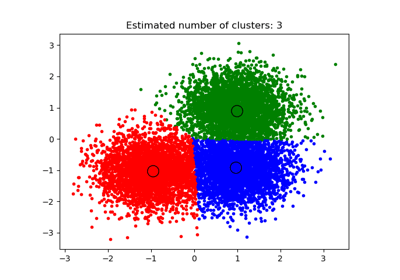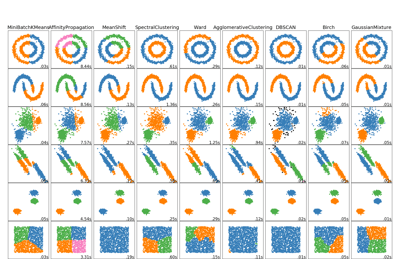/scikit-learn

# sklearn.cluster.MeanShift

`class sklearn.cluster.MeanShift(bandwidth=None, seeds=None, bin_seeding=False, min_bin_freq=1, cluster_all=True, n_jobs=None)` [source]

Mean shift clustering using a flat kernel.

Mean shift clustering aims to discover “blobs” in a smooth density of samples. It is a centroid-based algorithm, which works by updating candidates for centroids to be the mean of the points within a given region. These candidates are then filtered in a post-processing stage to eliminate near-duplicates to form the final set of centroids.

Seeding is performed using a binning technique for scalability.

Read more in the User Guide.

Parameters: `bandwidth : float, optional` Bandwidth used in the RBF kernel. If not given, the bandwidth is estimated using sklearn.cluster.estimate_bandwidth; see the documentation for that function for hints on scalability (see also the Notes, below). `seeds : array, shape=[n_samples, n_features], optional` Seeds used to initialize kernels. If not set, the seeds are calculated by clustering.get_bin_seeds with bandwidth as the grid size and default values for other parameters. `bin_seeding : boolean, optional` If true, initial kernel locations are not locations of all points, but rather the location of the discretized version of points, where points are binned onto a grid whose coarseness corresponds to the bandwidth. Setting this option to True will speed up the algorithm because fewer seeds will be initialized. default value: False Ignored if seeds argument is not None. `min_bin_freq : int, optional` To speed up the algorithm, accept only those bins with at least min_bin_freq points as seeds. If not defined, set to 1. `cluster_all : boolean, default True` If true, then all points are clustered, even those orphans that are not within any kernel. Orphans are assigned to the nearest kernel. If false, then orphans are given cluster label -1. `n_jobs : int or None, optional (default=None)` The number of jobs to use for the computation. This works by computing each of the n_init runs in parallel. `None` means 1 unless in a `joblib.parallel_backend` context. `-1` means using all processors. See Glossary for more details. `cluster_centers_ : array, [n_clusters, n_features]` Coordinates of cluster centers. labels_ : Labels of each point.

#### Notes

Scalability:

Because this implementation uses a flat kernel and a Ball Tree to look up members of each kernel, the complexity will tend towards O(T*n*log(n)) in lower dimensions, with n the number of samples and T the number of points. In higher dimensions the complexity will tend towards O(T*n^2).

Scalability can be boosted by using fewer seeds, for example by using a higher value of min_bin_freq in the get_bin_seeds function.

Note that the estimate_bandwidth function is much less scalable than the mean shift algorithm and will be the bottleneck if it is used.

#### References

Dorin Comaniciu and Peter Meer, “Mean Shift: A robust approach toward feature space analysis”. IEEE Transactions on Pattern Analysis and Machine Intelligence. 2002. pp. 603-619.

#### Examples

```>>> from sklearn.cluster import MeanShift
>>> import numpy as np
>>> X = np.array([[1, 1], [2, 1], [1, 0],
...               [4, 7], [3, 5], [3, 6]])
>>> clustering = MeanShift(bandwidth=2).fit(X)
>>> clustering.labels_
array([1, 1, 1, 0, 0, 0])
>>> clustering.predict([[0, 0], [5, 5]])
array([1, 0])
>>> clustering
MeanShift(bandwidth=2, bin_seeding=False, cluster_all=True, min_bin_freq=1,
n_jobs=None, seeds=None)
```

#### Methods

 `fit`(X[, y]) Perform clustering. `fit_predict`(X[, y]) Performs clustering on X and returns cluster labels. `get_params`([deep]) Get parameters for this estimator. `predict`(X) Predict the closest cluster each sample in X belongs to. `set_params`(**params) Set the parameters of this estimator.
`__init__(bandwidth=None, seeds=None, bin_seeding=False, min_bin_freq=1, cluster_all=True, n_jobs=None)` [source]
`fit(X, y=None)` [source]

Perform clustering.

Parameters: `X : array-like, shape=[n_samples, n_features]` Samples to cluster. `y : Ignored`
`fit_predict(X, y=None)` [source]

Performs clustering on X and returns cluster labels.

Parameters: `X : ndarray, shape (n_samples, n_features)` Input data. `y : Ignored` not used, present for API consistency by convention. `labels : ndarray, shape (n_samples,)` cluster labels
`get_params(deep=True)` [source]

Get parameters for this estimator.

Parameters: `deep : boolean, optional` If True, will return the parameters for this estimator and contained subobjects that are estimators. `params : mapping of string to any` Parameter names mapped to their values.
`predict(X)` [source]

Predict the closest cluster each sample in X belongs to.

Parameters: `X : {array-like, sparse matrix}, shape=[n_samples, n_features]` New data to predict. `labels : array, shape [n_samples,]` Index of the cluster each sample belongs to.
`set_params(**params)` [source]

Set the parameters of this estimator.

The method works on simple estimators as well as on nested objects (such as pipelines). The latter have parameters of the form `<component>__<parameter>` so that it’s possible to update each component of a nested object.

Returns: self

## Examples using `sklearn.cluster.MeanShift`A demo of the mean-shift clustering algorithmComparing different clustering algorithms on toy datasets